188-5712-5713

# 变频调速技术是如何推动桥梁技术的快速发展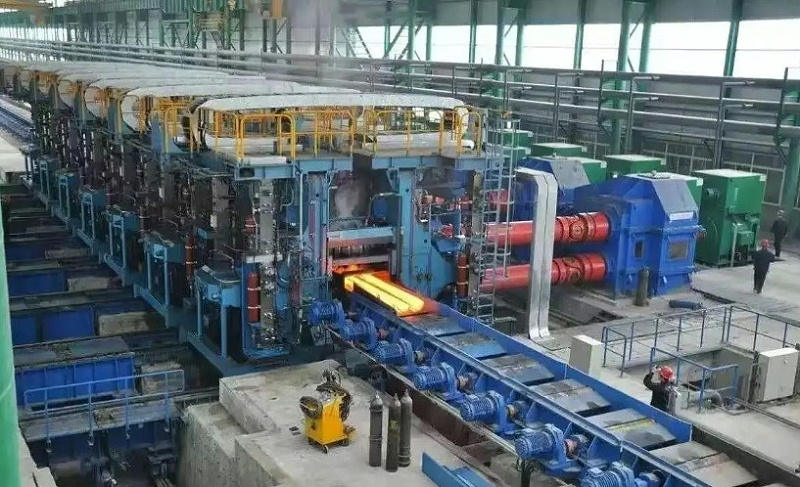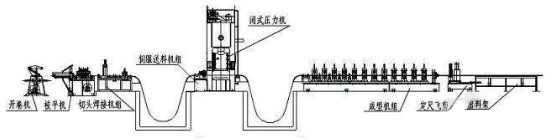(1)自调设置，F00.24=1，F15.01=37，F15.02=380，F15.03=68，F15.04=50，F15.05=1440，F15.06=2，F15.19=1。设定后。

(2)主机参数:F00.00=2，F01.00=1，F01.15=1，F02.11=1，F09.35=2，F09.36=17，F19.43=100

(3)从参数:F00.00=2，F01.15=1，F09.35=2，F09.36=16，F14.00=1，F14.13=002，F14.16=1，F14.17=1，F19.43=100

### 案例展示/ Hot CASE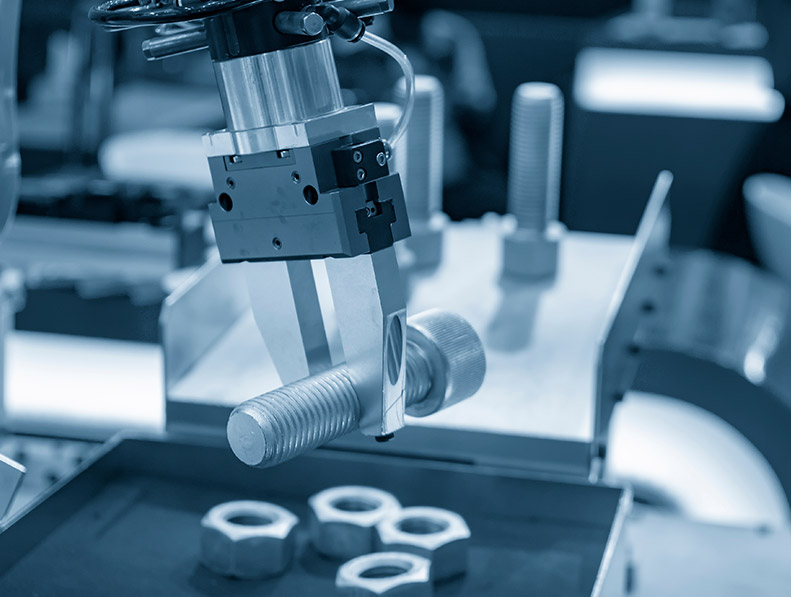#### 江苏某金属制品变频器节能改造案例！#### 数控机床变频器在河南数控设备中的应用案例！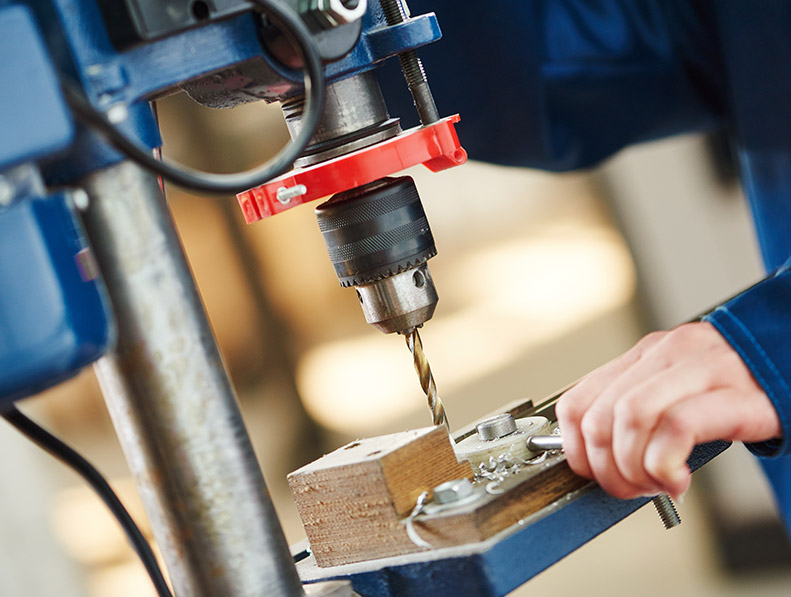#### SKI600系列变频器在杭州临安某机械设备生产企业中的应用案例！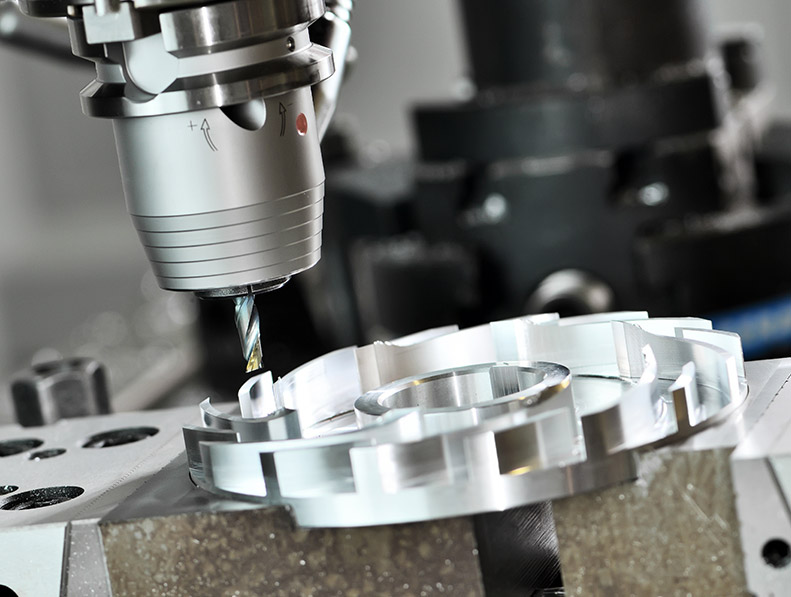## 三科变频资讯中心

### 客户案例

188-5712-5713
• 联系电话

188-5712-5713
• 微信扫一扫